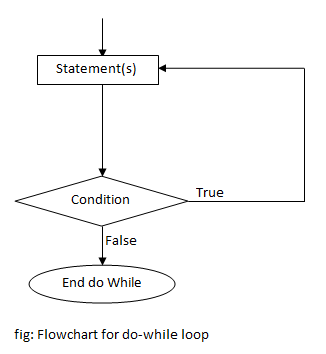# do-while loop in C++ Programming

In C++ programming, loop is a process of repeating a group of statements until a certain condition is satisfied. Do-while loop is a variant of while loop where the condition isn’t checked at the top but at the end of the loop, known as exit controlled loop. This means statements inside do-while loop are executed at least once and exits the loop when the condition becomes false or break statement is used. The condition to be checked can be changed inside the loop as well.

### Syntax of do-while loop

```do
{
statement(s);
... ... ...
}while (condition);```

### Flowchart of do-while loop### Example

C++ program to print the sum of n natural numbers.

```#include <iostream>
#include <conio.h>
using namespace std;

int main()
{
int n,i=1,s=0;
cout <<"Enter n:";
cin >> n;
do
{
s=s+i;
i++;
}while (i<=n);
cout <<"Sum = "<<s;
getch();
return 0;
}```

This program prints the sum of first n natural numbers. The number till which the sum is to be found is asked from user and stored in a variable n. The variables i and s are used to store the number count from 1…n and sum of the numbers respectively. Inside the do-while loop, sum is calculated by repeated addition and increment. In each repetition, whether number count, i, is smaller or equals to inputted number, n, is checked. If it is, then the loop continues, but exits the loop if it isn’t. After the control exits from the loop, sum is printed.

Output

```Enter n:7
Sum = 28```